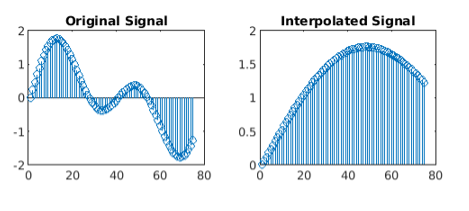# Up-sampling in MATLAB

Interpolation or up-sampling is the specific inverse of decimation. It is a data saving operation, in that all examples of x[n] are available in the extended signal y[n]. Interpolation works by adding (L–1) zero-valued examples for each input sample.

We will be using the interp() function to interpolate a signal. It is used to increase the sample rate of a signal by an integer factor.

Syntax: a = interp(x, r)
Parameter:

• x: input signal
• r: interpolation factor

Return Value: Returns interpolated signal

MATLAB code for interpolation of a signal:

## MATLAB

 `% time vector ` `t = 0 : .00025 : 1; ` ` `  `# input signal ` `x = sin(2 * pi * 50 * t) + sin(2 * pi * 100 * t); ` ` `  `% increase the sample rate of i/p signal by factor of 4 ` `y = interp(x, 4);  ` ` `  `subplot(2, 2, 1); ` ` `  `% plot few samples of the Original signal ` `stem(x(1 : 75))  ` `title(``'Original Signal'``); ` ` `  `subplot(2, 2, 2); ` ` `  `% plots few samples of the up-sampled signal ` `stem(y(1 : 75));  ` `title(``'Interpolated Signal'``); `

Output:Attention reader! Don’t stop learning now. Get hold of all the important CS Theory concepts for SDE interviews with the CS Theory Course at a student-friendly price and become industry ready.

My Personal Notes arrow_drop_upCheck out this Author's contributed articles.

If you like GeeksforGeeks and would like to contribute, you can also write an article using contribute.geeksforgeeks.org or mail your article to contribute@geeksforgeeks.org. See your article appearing on the GeeksforGeeks main page and help other Geeks.

Please Improve this article if you find anything incorrect by clicking on the "Improve Article" button below.

Article Tags :

1

Please write to us at contribute@geeksforgeeks.org to report any issue with the above content.